# Cornish-Fisher expansion

(diff) ← Older revision | Latest revision (diff) | Newer revision → (diff)

An asymptotic expansion of the quantiles of a distribution (close to the normal standard one) in terms of the corresponding quantiles of the standard normal distribution, in powers of a small parameter. It was studied by E.A. Cornish and R.A. Fisher . If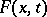is a distribution function depending onas a parameter, if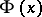is the normal distribution function with parameters, and if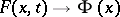as, then, subject to certain assumptions on, the Cornish–Fisher expansion of the function(where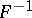is the function inverse to) has the form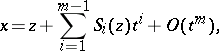(1)

where the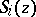are certain polynomials in. Similarly, one defines the Cornish–Fisher expansion of the function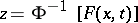(being the function inverse to) in powers of:(2)

where the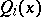are certain polynomials in. Formula (2) is obtained by expandingin a Taylor series about the pointand using the Edgeworth expansion. Formula (1) is the inversion of (2).

Ifis a random variable with distribution function, then the variable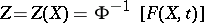is normally distributed with parameters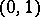, and, as follows from (2),approximates the distribution function of the variable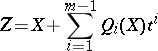as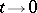better than it approximates. Ifhas zero expectation and unit variance, then the first terms of the expansion (1) have the form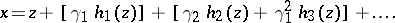Here,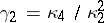, withthe-th cumulant of,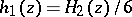,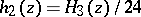,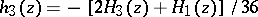, and withthe Hermite polynomials, defined by the relation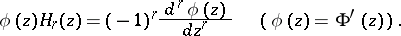Concerning expansions for random variables obeying limit laws from the family of Pearson distributions see . See also Random variables, transformations of.

How to Cite This Entry:
Cornish-Fisher expansion. Encyclopedia of Mathematics. URL: http://encyclopediaofmath.org/index.php?title=Cornish-Fisher_expansion&oldid=14424
This article was adapted from an original article by V.I. Pagurova (originator), which appeared in Encyclopedia of Mathematics - ISBN 1402006098. See original article